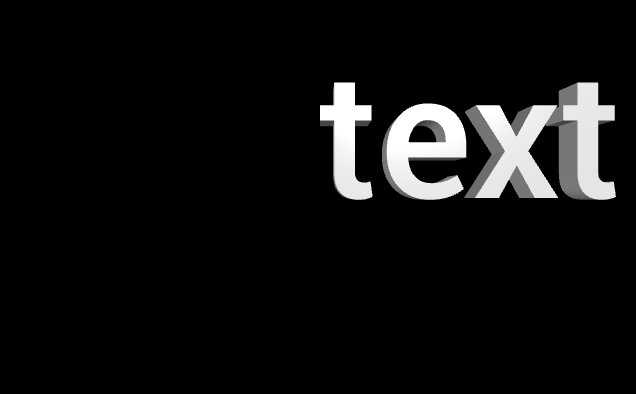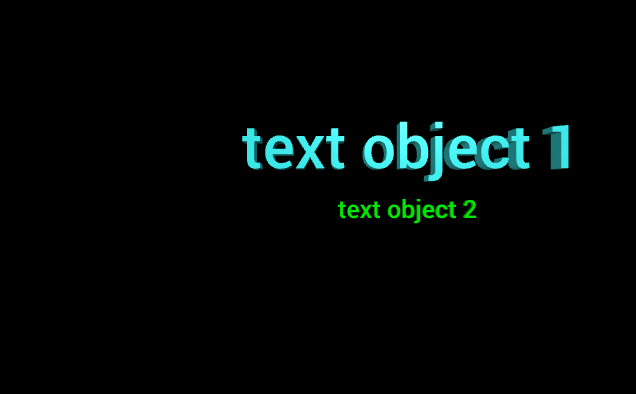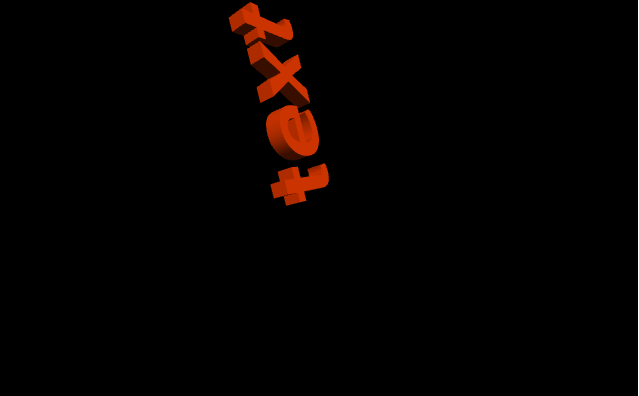# Making a text object with VPython

`VPython` makes it easy to create navigable 3D displays and animations, even for those with limited programming experience. Because it is based on Python, it also has much to offer for experienced programmers and researchers. `VPython` allows users to create objects such as spheres and cones in 3D space and displays these objects in a window. This makes it easy to create simple visualizations, allowing programmers to focus more on the computational aspect of their programs. The simplicity of `VPython` has made it a tool for the illustration of simple physics, especially in the educational environment.

Installation :

`pip install vpython`

A text object is used to display 3D textual data. We can generate a text object in `VPython` using the `text()` method.

## text() method

Syntax : text(parameters)

Parameters :

• pos : It is the position of the text object. Assign a vector containing 3 values, example pos = vector(0, 0, 0)
• align : It is the alignment of the text obect. Assign a string with either of the options, “center”, “right” and “left”, default is “left”
• height : It is the height of an uppercase letter. Assign a floating value, the default is 1, example height = 18
• length : It is the length of the displayed text. Assign a floating value, example length = 4
• depth : It is the depth of the displayed text. Assign a floating value, the default is 0.2 * height, example depth = 2
• axis : It is the axis of alignment of the text object. Assign a vector containing 3 values, example axis = vector(1, 2, 1)
• up : It is the orientation of the text object. Assign a vector containing 3 values, example up = vector(0, 1, 0)
• font : It is the font of the text. Assign a string with values either “sans or “serif”
• color : It is the color of the text. Assign a vector containing 3 values, example color = vector(1, 1, 1) will give the color white
• background : It is the color of the background of the label. Assign a vector containing 3 values, example color = vector(1, 1, 1) will give the background color white
• billboard : It determines whether the text object always faces you or not. Assign a boolean value in which True is yes and False is no
• opacity : It is the opacity of the text object. Assign a floating value in which 1 is the most opaque and 0 the least opaque, example opacity = 0.5
• shininess : It is the shininess of the text object. Assign a floating value in which 1 is the most shiny and 0 the least shiny, example shininess = 0.6
• emissive : It is the emissivity of the text object. Assign a boolean value in which True is emissive and False is not emissive, example emissivity = False
• text : It is the text to be displayed. HTML styles can also be included while assigning the text.
• descender : It is the height of the descender on lower-case letters such as y. Assign a floating value, the default is 0.3 * height, example descender = 8
• upper_left, upper_right, lower_right, lower_left : They are the bounding box of the displayed text
• start, end : They are the left-most and right-most locations on the baseline
• vertical_spacing : It is the vertical distance from one baseline to the next in a multiline text

All the parameters are optional.

Example 1 :A text object with only the text parameter, all the other parameters will have the default value.

 `# import the module ` `from` `vpython ``import` `*` `text(text ``=` `"text"``) `

Output :Example 2 :A text object using the parameters color, opacity, shininess and emissivity.

 `# import the module ` `from` `vpython ``import` `*` `text(text ``=` `"text"``, ` `     ``color ``=` `vector(``0``, ``0``, ``1``),  ` `     ``opacity ``=` `0.5``,  ` `     ``shininess ``=` `1``,  ` `     ``emissive ``=` `False``) `

Output :Example 3 :Displaying 2 text objects to visualize the attributes pos, height and depth.

 `# import the module ` `from` `vpython ``import` `*` ` `  `# the first text object ` `text(text ``=` `"text object 1"``, ` `     ``pos ``=` `vector(``-``5``, ``2``, ``0``), ` `     ``height ``=` `3``, ` `     ``depth ``=` `1``, ` `     ``color ``=` `vector(``0.5``, ``1``, ``1``)) ` `  `  `# the second text object ` `text(text ``=` `"text object 2"``, ` `     ``pos ``=` `vector(``1``, ``-``1``, ``5``),  ` `     ``color ``=` `vector(``0``, ``1``, ``0``)) `

Output :Example 4 :A cylinder using the parameters axis and up.

 `# import the module ` `from` `vpython ``import` `*` `text(text ``=` `"text"``, ` `     ``color ``=` `vector(``1``, ``0.5``, ``0``), ` `     ``axis ``=` `vector(``-``1``, ``4``, ``0``), ` `     ``up ``=` `vector(``1``, ``2``, ``2``)) `

Output :My Personal Notes arrow_drop_upCheck out this Author's contributed articles.

If you like GeeksforGeeks and would like to contribute, you can also write an article using contribute.geeksforgeeks.org or mail your article to contribute@geeksforgeeks.org. See your article appearing on the GeeksforGeeks main page and help other Geeks.

Please Improve this article if you find anything incorrect by clicking on the "Improve Article" button below.

Article Tags :

Be the First to upvote.

Please write to us at contribute@geeksforgeeks.org to report any issue with the above content.